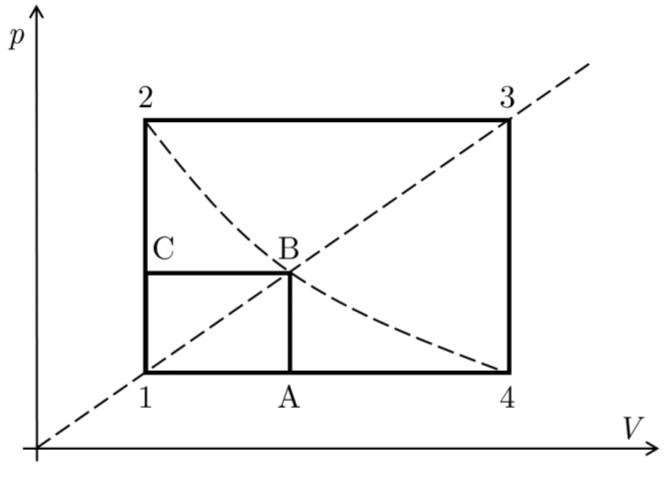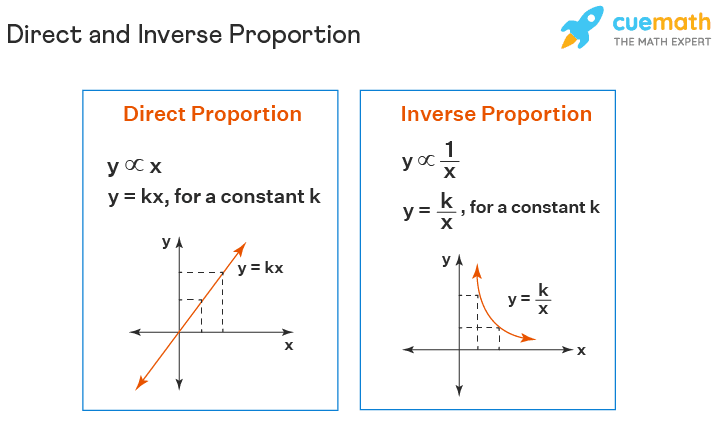# Calculate these 5 temperatures along this Thermodynamic cycle

• Seeit

#### Seeit

Homework Statement
Calculate the temperatures at places 2, 4, A, B and C if you know:
It's an ideal diatomic gas
T3 = 4T1
T2 = Tb = T4
The axis connecting 1, B and 3 crosses zero.
Relevant Equations
pV = nRT
Laws of thermodynamicsI only know T3 = 4•T1
I was able to calculate the T2 = Tb = T4
I built four equations:
T2 = p2V1 / nR
T4 = p1V2 / nR
p1/T1 = p2/T2
V1/T2 = V2/4T1

I put them together and got T2 = 2T1

I can't figure out the temperatures of A and C. I tend to think Ta could equal Tc (then I would be able to calculate it), but I am not sure.

Last edited:

Helo @Seeit ,##\qquad## !​
Are you sure you have rendered the complete problem statement ? I would expect some more information, like: ideal gas, isothermal (or adiabatic), ...

I also miss an equation of state in your relevant equations (e.g. ##\ pV = NRT##).

I only know ##T_3 = 4T_1##
How ? Or was that a given ? (In that case it is part of the problem statement)
Same for ##T_2 = T_b = T_4## ?

What about the scale and the axes of the diagram ?

##\ ##

•Seeit and Lnewqban
Welcome, @Seeit !

As post #1 has been edited to answer @BvU questions, I suggest considering two things:

-The inverse proportionality between p and V.
-The similarity between polygons 1CBA and 1234 due to the axis connecting 1, B and 3, which makes their corresponding sides proportional.•Seeit and BvU
Welcome, @Seeit !

As post #1 has been edited to answer @BvU questions, I suggest considering two things:

-The inverse proportionality between p and V.
-The similarity between polygons 1CBA and 1234 due to the axis connecting 1, B and 3, which makes their corresponding sides proportional.

View attachment 324057
So am I right about thinking that A and C are on the same isotherm and have therefore the same temperature?

So am I right about thinking that A and C are on the same isotherm and have therefore the same temperature?
Correct!

•Seeit